# Test: Real Analysis- 8

## 20 Questions MCQ Test Topic-wise Tests & Solved Examples for IIT JAM Mathematics | Test: Real Analysis- 8

Description
This mock test of Test: Real Analysis- 8 for Mathematics helps you for every Mathematics entrance exam. This contains 20 Multiple Choice Questions for Mathematics Test: Real Analysis- 8 (mcq) to study with solutions a complete question bank. The solved questions answers in this Test: Real Analysis- 8 quiz give you a good mix of easy questions and tough questions. Mathematics students definitely take this Test: Real Analysis- 8 exercise for a better result in the exam. You can find other Test: Real Analysis- 8 extra questions, long questions & short questions for Mathematics on EduRev as well by searching above.
QUESTION: 1

### Let R and S be two non-void relations on a set A. Q. Which of the following statements is false?

Solution:

Let A = {1, 2, 3} and R = {(1, 1), (1, 2)}
S = {(2, 2), (2, 3)} be transitive relations on A.
Then, R ∪ S= {(1,1); (1,2); (2,2); (2, 3)} Obviously, R ∪ S is transitive.
Since, (1, 2) ∈ R ∪ S and (2, 3 ) ∈ R ∪ S but (l , 3) ∉ R ∪ S.

QUESTION: 2

### Consider the following statements For non-empty sets A, B and C 1. A - (B - C) = (A - B) ∪ C 2. A - (B - C) = (A - B) - C ​Q. Which of the statements given above is/are correct?

Solution:

In the given statements, statement (I) is correct.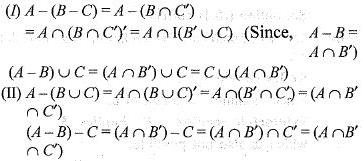QUESTION: 3

### Let N be the set of integers. A relation R on N is defined as R = {(x, y) | xy > 0, x, y ∈ N}. Then, which one of the following is correct?

Solution:

Since, R = {(x, y) | xy > 0, x, y ∈ N}

Reflexive
Since, x, y ∈ N
So, x, x ∈ N
implies x2 > 0
Hence, R is reflexive.
Symmetric
Since, x, y ∈ N and xy > 0 implies yx > 0 Hence, R is also symmetric.
Transitive
Since, x,y, z, ∈ N
implies xy > 0, yz > 0
implies xz > 0
So, R is also transitive
Thus, R is an equivalence relation.

QUESTION: 4

Which one of the following function f : R → R is injective?

Solution:

An injective function means one-one.
In option (d),f (x) = -x
For every values of x, we get a different values of f.
Hence, it is injective.

QUESTION: 5

If A is the set of even natural number less than 8 and B is the set of prime number less than 7, then number of relations from A to B is

Solution:

A = {2,4,6}, B = {2,3, 5}
So, A x B contains 3 x 3 = 9 elements.
Hence, number of relations from A to B = 29.

QUESTION: 6

What does the shaded region in the Venn diagram given following represent?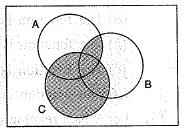Solution:

The shaded region in the Venn diagram is represented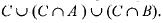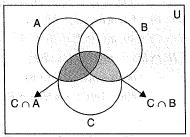QUESTION: 7

Out of 32 persons, 30 invest in National savings Certificates and 17 invest in shares. What is the number of persons who invest in both?

Solution:

n(N ∪ S) = 32, n(N) = 30, n(S) = 17 Since, We know that,
n( N ∪ S) = n(N) + n(S) - n(N ∩ 5)
or  32= 30+ 17 - n(N ∩ S)
or n(N ∩ S ) = 47 - 32= 15
Since, R is an equivalence relation on set A, Therefore, (a, a) ∈ R for all a ∈ A, Hence, R has atleast n ordered pairs.

QUESTION: 8

Let R = {x | x ∈ N ,x is multiple of 3 and x ≤ 10} and S = {x | x ∈ N, x is a multiple of 5 and x ≤ 100} What is the number of elements in (R x S) ∩ (S x R) ?

Solution:

Since, R = {3,6,9,12,15,..., 99}
and S= {5,10,15, ...,95}
Now, (R x S) ∩ ( S x  R)
= (R ∩ S ) x ( S ∩ R ) = (15,30,45,60,75,90) x (15,30, 45,60,75,90)
Hence, number of elements in
(R x S) ∩ (S x R) = 6 x 6 = 36

QUESTION: 9

Let X be the set of all graduates in India. Elements x and y in X are said to be related, if they are graduates of the same university. Which one of the following statements is correct?

Solution:

xRy ⇒ x and y are graduates of the same university.
Reflexive xRx ⇔ x and y are graduates of the same university.
So, relation is reflexive.
Symmetric xRy ⇔ x and y are graduates of the same university.
implies, yRx ⇔ y and x are graduates of the same university.
So, relation is symmetric.
Transitive xRy, yRz ⇔ xRz
It means x and y, y and z are graduates of the same university, then x and z are also graduates of the same university.
So, relation is transitive.
Hence, relation is reflexive, symmetric and transitive.

QUESTION: 10

In a college of 300 students, every student reads 5 newspaper and every newspaper is read by 60 students. The number of newspaper is

Solution:

Let number of newspapers be x.
If every students reads one newspaper, the number of students would be
x(60) = 60x
Since, every students reads 5 newspapers
Hence, number of students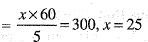QUESTION: 11

Out of 800 boys in a school, 224 played cricket, 240 played hockey and 336 played basketball. Of the total, 64 played both basketball and hockey; 80 played cricket and basketball and 40 played cricket and hockey; 24 played all the three games. The number of boys who did not play any game is

Solution:

Here,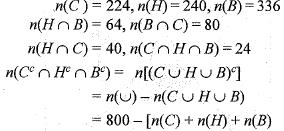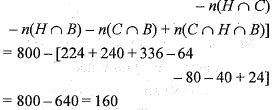QUESTION: 12

Let A = {-1, 2, 5, 8}, B = {0, 1, 3, 6, 7} and R be the relation ‘is one less than’ from A to B, then how many elements will R contain?

Solution:

Given,
A = { - 1 , 2 , 5, 8} and
B = {0 ,1 ,3 ,6 ,7 }
R = { ( - 1 , 0 ) , (2,3), (5,6)}
(Since , R = A is one less than from B) Hence, total number of elements in R is 3.

QUESTION: 13

Consider the function f:R → {0, 1} such thatQ. Which one of the following is correct?

Solution:

Since, on taking a straight line parallel to x-axis, the group of given function intersect it at many points.
So, f(x) is a many one.
And as range of f(x) = codomain
So, f(x) is onto.
Hence, f(x) is many one onto.

QUESTION: 14

Let R be a relation over the set N x N and it is defined by (a, b)R (c, d) ⇒ a + d = b + c. Then R is

Solution:

We have (a,b) R (a, b) for all (a, b) ∈ N x N
Since. a + b = b + a.
Hence, R is reflexive.
R is symmetric for we have (a , b) R (c, d)
implies a + d = b + c
implies d + a = c + b
implies c + b = d + a
implies (c, d) R (e, f)
Then, by definition of R,
we have a + d = b + c and c + f = d + e when by addition, we get
a + d + c + f = b + c + d + e
or a + f = b + e
Hence, (a, b) R (e , f)
Thus, (a, b) R( c, d) and (c, d) R (e , f) implies (a , b) R (e , f)

QUESTION: 15

Let N denote the set of natural numbers and A = {n2 : n ∈ N} and B = {n3/2 : n ∈ N}. Which one of the following is correct?

Solution:

Since, A = { n2 : n ∈ N } and
B = {n3 : n ∈ N}
A = { 1 ,4 ,9 ,1 6 ,2 5 ,4 9 ,6 4 ,8 1 ,...}
B = {1 ,8 ,2 7 ,6 4 ,1 2 5 ,...}
A ∩ B = { 1 , 6 4 , . .....}
A ∩ B must be a proper subset of {m6 : m ∈ N}

QUESTION: 16

If A and. B are two disjoint sets, then which one of the following is correct?

Solution:

Since, A ∩ B = φ (given)
So, A - B = A - ( A ∩ B )
(Since, A - B = A)
Now, B - A' = φ
and B ∩ A = φ
or B ‘- A ' = B ∩ A
and (A- B ) ∩ B = A ∩ B
So, option (d) is correct.

QUESTION: 17

Let M be the set of men and R is a relation ‘is son of’ defined on M. Then, R is

Solution:

M = set of men and R is a relation ‘is son of ’ defined on M.
Reflexive relation aRa.
Since, a cannot be a son o f a.
Symmetric relation aRimplies bRa which is also not possible.
Transitive relation aRb, bRc implies cRwhich is not possible.

QUESTION: 18

Let f : R --> R be a function defined a s / ( x ) = x | x |; for each x ∈ R, R being the set of real numbers. Which one of the following is correct?

Solution:

Since, f(x) = x |x|
If f(x1) = f(x2)
or x1 | x1| = x| x2 |
or x1 = x2
So, f(x) is one-one.
Also, range of f(x) = codomain of f(x)
So, f(x) is onto.
Hence, f(x) is both one-one and onto.

QUESTION: 19

What is the range of f(x) = cos 2x - sin 2x?

Solution:

Since, f(x) = cos 2x - sin 2x
[Since, f( x ) = a cos x + b sin x,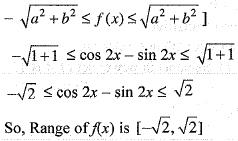QUESTION: 20

Consider the following with regard to a relation R on a set of real number defined by xRy if and only if 3x + 4y = 5.
1. 0R
2. 1R1/2
3. 2/3R3/4
Q. Which of the above are correct?

Solution:

Since, on the set of real numbers, R is a relation defined by XRy if and only if 3x + 4y = 5 for which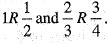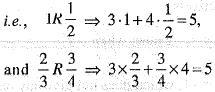Hence, both the statements 11 and 111 are correct.